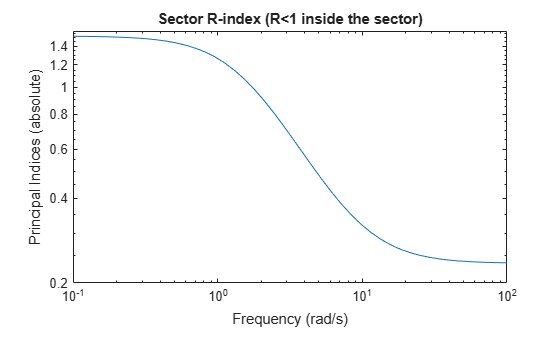# getSectorCrossover

Crossover frequencies for sector bound

## Syntax

``wc = getSectorCrossover(H,Q)``

## Description

example

````wc = getSectorCrossover(H,Q)` returns the frequencies at which the following matrix M(ω) is singular:$M\left(\omega \right)=H{\left(j\omega \right)}^{H}Q\text{\hspace{0.17em}}H\left(j\omega \right).$When a frequency-domain sector plot exists, these frequencies are the frequencies at which the relative sector index (R-index) for `H` and `Q` equals 1. See About Sector Bounds and Sector Indices for details.```

## Examples

collapse all

Find the crossover frequencies for the dynamic system $G\left(s\right)=\left(s+2\right)/\left(s+1\right)$ and the sector defined by:

`$S=\left\{\left(y,u\right):a{u}^{2}`

for various values of a and b.

In U/Y space, this sector is the shaded region of the following diagram (for a, b > 0).The `Q` matrix for this sector is given by:

`$Q=\left[\begin{array}{cc}1& -\left(a+b\right)/2\\ -\left(a+b\right)/2& ab\end{array}\right];\phantom{\rule{1em}{0ex}}a=0.1,\phantom{\rule{0.2777777777777778em}{0ex}}b=10.$`

`getSectorCrossover` finds the frequencies at which $H\left(s{\right)}^{H}QH\left(s\right)$ is singular, for $H\left(s\right)=\left[G\left(s\right);I\right]$. For instance, find these frequencies for the sector defined by `Q` with a = 0.1 and b = 10.

```G = tf([1 2],[1 1]); H = [G;1]; a = 0.1; b = 10; Q = [1 -(a+b)/2 ; -(a+b)/2 a*b]; w = getSectorCrossover(H,Q)```
```w = 0x1 empty double column vector ```

The empty result means that there are no such frequencies.

Now find the frequencies at which ${H}^{H}QH$ is singular for a narrower sector, with a = 0.5 and b = 1.5.

```a2 = 0.5; b2 = 1.5; Q2 = [1 -(a2+b2)/2 ; -(a2+b2)/2 a2*b2]; w2 = getSectorCrossover(H,Q2)```
```w2 = 1.7321 ```

Here the resulting frequency is where the R-index for `H` and `Q2` is equal to 1, as shown in the sector plot.

`sectorplot(H,Q2)`Thus, when a sector plot exists for a system `H` and sector `Q`, `getSectorCrossover` finds the frequencies at which the R-index is 1.

## Input Arguments

collapse all

Model to analyze against sector bounds, specified as a dynamic system model such as a `tf`, `ss`, or `genss` model. `H` can be continuous or discrete. If `H` is a generalized model with tunable or uncertain blocks, `getSectorCrossover` analyzes the current, nominal value of `H`.

To get the frequencies at which the I/O trajectories (u,y) of a linear system G lie in a particular sector, use ```H = [G;I]```, where `I = eyes(nu)`, and `nu` is the number of inputs of `G`.

Sector geometry, specified as:

• A matrix, for constant sector geometry. `Q` is a symmetric square matrix that is `ny` on a side, where `ny` is the number of outputs of `H`.

• An LTI model, for frequency-dependent sector geometry. `Q` satisfies Q(s)’ = Q(–s). In other words, Q(s) evaluates to a Hermitian matrix at each frequency.

The matrix `Q` must be indefinite to describe a well-defined conic sector. An indefinite matrix has both positive and negative eigenvalues.

## Output Arguments

collapse all

Sector crossover frequencies, returned as a vector. The frequencies are expressed in rad/`TimeUnit`, relative to the `TimeUnit` property of `H`. If the trajectories of `H` never cross the boundary, `wc = []`.